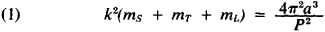Gaussian Constant

Gaussian constant

[¦gau̇·sē·ən ′kän·stənt]
(astronomy)
The acceleration caused by the attraction of the sun at the mean distance of the earth from the sun.
McGraw-Hill Dictionary of Scientific & Technical Terms, 6E, Copyright © 2003 by The McGraw-Hill Companies, Inc.
The following article is from The Great Soviet Encyclopedia (1979). It might be outdated or ideologically biased.

Gaussian Constant

one of the fundamental astronomical constants (designated k). It was first defined by K. Gauss as the approximate value of the square root of the gravitational constant k2, which is found in the formula of the two-body problem (in celestial mechanics):This formula relates the sun’s mass ms, the earth’s mass mT, and the moon’s mass mL to the period of revolution P of the earth-moon system along an elliptical orbit around the sun and to the semimajor axis a of this orbit. Gauss took the mass of the sun and the indicated semimajor axis a as the units of mass and length and the mean solar day as the unit of time. Using the values of P and the ratios mT/ms and mL/mT current in his day, Gauss found

k = 0.01720209895

This value of k (which is considered accurate) is part of the modern system of fundamental astronomical constants and is called the Gaussian constant. Provided that the ephemeris day is used as the unit of time, the unit of distance corresponding to this value of k and to formula (1) is called the astronomical unit (a.u.). This differs somewhat from the semimajor axis a of the orbit of the earth-moon system, which according to formula (1) and the contemporary values of P, mT/mS, and mL/mT is 1.000000032 a.u.

IU. A. RIABOV